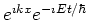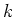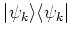Previous: 7.2.1 Separation of Neumann Up: 7.2 The Open Von Next: 7.2.3 Self Consistency

## 7.2.2 Open System

In our application the environment (electrodes) absorbs outgoing particles and injects particles into the device with a Fermi-Dirac equilibrium distribution which is fixed by the demand of local charge neutrality at the boundary. Adding boundary conditions can change the character of the model and in general a separation ansatz will no longer work. However, if we have an open system and incoming waves are mixed states built from plane waves of the formthen this type of boundary conditions can still be treated by the separation approach.

In this way the von Neumann equation with absorbing boundary conditions is equivalent to a countable system of Schrödinger equations with transparent boundary conditions. In practice we solve the open Schrödinger equation for a set of values for. Then the solution is built from the pure stateswhich are summed with the correct weight given by the equilibrium Fermi-Dirac distribution in the electrodes. Solving the von Neumann equation by the separation method is known as the quantum transmitting boundary method. It has been introduced in [LK90b]. It has been applied for device simulations in [BA00], and [Fre87]. For an extension see [LKF02].

The reflection and transmission coefficients for pairs of energy-related incoming waves from left and right are not independent [Akt99] which can be used to reduce the numerical costs by a factor of 2.

Ballistic transport is only a good approximation if the influence of scattering can be neglected. The catch here is that in such cases the assumption of injection with an equilibrium distribution is doubtful.